# Finding the current through the battery

• Vanessa Avila
In summary, the student is trying to find the equivalent resistance for a battery with a 14V load and a horizontal 1 ohm resistor. They are unsure what to do because they believe their required resistance is incorrect. They are also confused about the current through the battery and have a backup plan.

## Homework Statement

I am confused what to do but I have to find the current through the battery: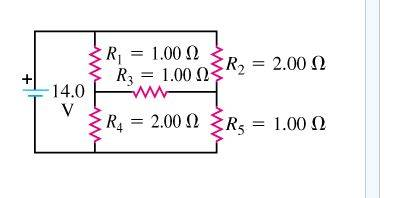V=IR

## The Attempt at a Solution

I decided to find the equivalent resistance and do V/R = I using the 14V, but I believe my Req is wrong. What is throwing me off is the horizontal 1 ohm resistor.

Show the details of your attempt. How did you try to reduce the network to a single equivalent resistance?

What's your backup plan?Vanessa Avila said:

## Homework Statement

I am confused what to do but I have to find the current through the battery:V=IR

## The Attempt at a Solution

I decided to find the equivalent resistance and do V/R = I using the 14V, but I believe my Req is wrong. What is throwing me off is the horizontal 1 ohm resistor.
There may be a trick to simplify it because of the symmetry of the resistance values, but it's probably just easiest to write the KCL equations and solve them. Can you show us that work?

EDIT -- Oops, beaten out by gneill again!Would this be right?

14V - I1(1Ω) - I1(2Ω) = 0
So that would give me I1 = 4.67 A

Vanessa Avila said:
Would this be right?

14V - I1(1Ω) - I1(2Ω) = 0
So that would give me I1 = 4.67 A
You'll have to show us what ##i_1## is on your diagram, but I would say that it's very unlikely. Any current through R3 would "break" any attempt to consider the vertical paths as isolated series connected resistors.

gneill said:
You'll have to show us what ##i_1## is on your diagram, but I would say that it's very unlikely. Any current through R3 would "break" any attempt to consider the vertical paths as isolated series connected resistors.
My bad. Here's what I was trying to do: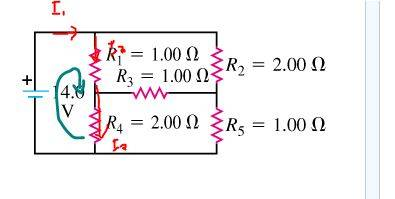I realized that the I1 splits as soon as it reaches the junction and i can either keep going to the right or down. So that means my equation for my loop has to involve I2 right?

Vanessa Avila said:
My bad. Here's what I was trying to do:I realized that the I1 splits as soon as it reaches the junction and i can either keep going to the right or down. So that means my equation for my loop has to involve I2 right?
You can either write the multiple KVL equations, or the multiple KCL equations. I personally prefer KCL, but either will work. Are you familiar with how to write them?

berkeman said:
You can either write the multiple KVL equations, or the multiple KCL equations. I personally prefer KCL, but either will work. Are you familiar with how to write them?
Yes. How my professor taught it to us is that we have to set directions for the current and create a clockwise or a counter-clockwise loop. My biggest problem with it is keeping it to only 3 unknown currents (I1, I2, and I3) as he advised. I get confused when I am past assigning where I3 is.

I think how i labeled the diagram was wrong. Would the current going through R3 be (I2 + I3) ?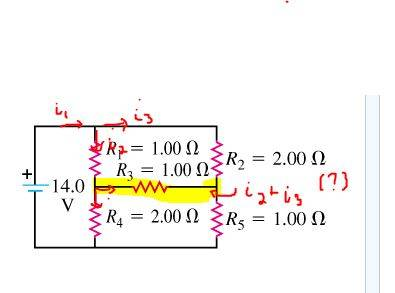One way to minimize the number of currents you "invent" while labeling the circuit is to only ever add the minimum required number of currents at any junction you come across while proceeding systematically through the circuit. If you can, label new paths using mathematical combinations of existing currents.

For example, if you go into a junction with i1 and two unlabeled paths diverge from there, make one current i2 and the other i1-i2. Thus only one "new" current is introduced at that junction rather than two.

•Vanessa Avila
gneill said:
One way to minimize the number of currents you "invent" while labeling the circuit is to only ever add the minimum required number of currents at any junction you come across while proceeding systematically through the circuit. If you can, label new paths using mathematical combinations of existing currents.

For example, if you go into a junction with i1 and two unlabeled paths diverge from there, make one current i2 and the other i1-i2. Thus only one "new" current is introduced at that junction rather than two.

Okay. I relabeled everything. Can you check to see if i did it right?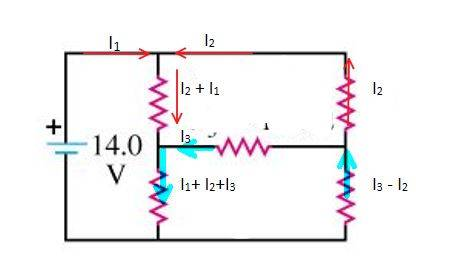Check the KCL sum at the center right node. Everything else looks okay.

gneill said:
Check the KCL sum at the center right node. Everything else looks okay.
would it be -I3-I2?

Vanessa Avila said:
would it be -I3-I2?
No. remember, whatever is flowing out of the node must also be flowing into the node. You have I2 and I3 both flowing out, so what must flow in on the remaining path?

I2 + I3 ?

Sorry, I'm a little confused about this stuffVanessa Avila said:
I2 + I3 ?
Yup.
Sorry, I'm a little confused about this stuffNo worries. After a bit of practice it'll suddenly "cick" and you'll wonder what all the fuss was aboutgneill said:
Yup.

No worries. After a bit of practice it'll suddenly "cick" and you'll wonder what all the fuss was aboutOh okay! ThanksSo if I were to assign three loops like this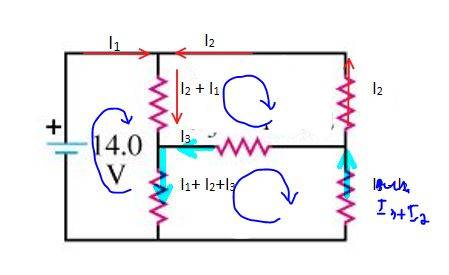would these be the right eqns?

Eqn #1: (loop with 14V, starting after the 14V)
14V - (I2+I1)*(1Ω) - (I1+I2+I3)*(2Ω) = 0

Eqn #2: (upper loop, starting above I2+I1)
(I2+I1)*(1Ω)+(I2)*(2Ω) - (I3)*(1Ω) = 0

Eqn #3: (lower loop, starting above I1+I2+I3)
(I1+I2+I3)*(2Ω) + I3*(1Ω) + (I3+I2)*(1Ω) = 0

I'd say that they look good. I don't spot any problems.

gneill said:
I'd say that they look good. I don't spot any problems.
:) Awesome! So finding the current through the battery, i have to find I1, I2 and I3 and sum them together to use in the V=IR equation, where V = 14V?

Vanessa Avila said:
:) Awesome! So finding the current through the battery, i have to find I1, I2 and I3 and sum them together to use in the V=IR equation, where V = 14V?
Your I1 flows through the battery. Find I1 and you're done (of course you need to mostly solve everything to get there... unless you use something like Cramer's Rule to find just the one current).

•Vanessa Avila
Okay thanks a lot! :) I really appreciate it!
gneill said:
Your I1 flows through the battery. Find I1 and you're done (of course you need to mostly solve everything to get there... unless you use something like Cramer's Rule to find just the one current).